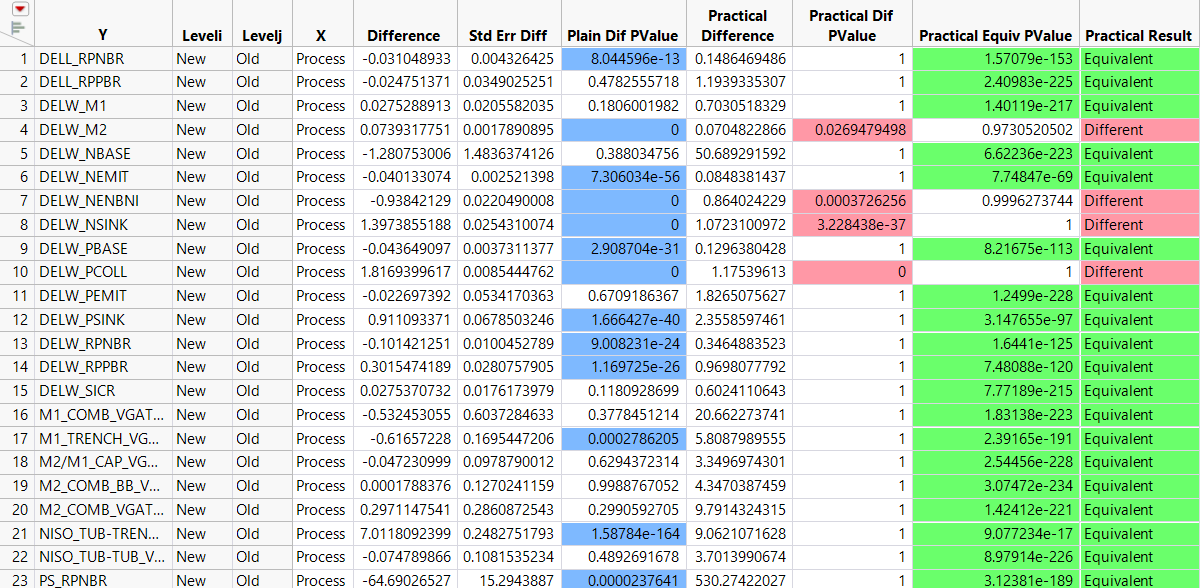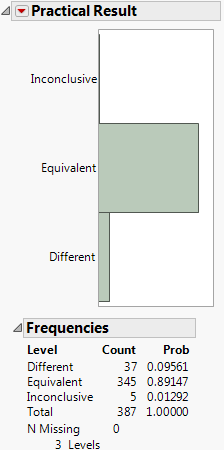Predictive and Specialized Modeling > Response Screening > Additional Examples of Response Screening > Example of Tests of Practical Significance and Equivalence
Publication date: 07/30/2020

## Example of Tests of Practical Significance and Equivalence

This example tests for practical differences using the Probe.jmp sample data table.

1. Select Help > Sample Data Library and open Probe.jmp.

2. Select Analyze > Screening > Response Screening.

The Response Screening Launch window appears.

3. Select the Responses column group and click Y, Response.

4. Select Process and click X.

5. Type 0.15 in the Practical Difference Portion box.

6. Click OK.

7. Click the Response Screening red triangle and select Save Compare Means.

Figure 21.12 shows a portion of the data table. For each response in Y, the corresponding row gives information about tests of the New and the Old levels of Process.

Figure 21.12 Compare Means Table, Partial ViewBecause specification limits are not saved as column properties in Probe.jmp, JMP calculates a value of the practical difference for each response. The practical difference of 0.15 that you specified is multiplied by an estimate of the 6σ range of the response. This value is used in testing for practical difference and equivalence. It is shown in the Practical Difference column.

The Plain Difference column shows responses whose p-values indicate significance. The Practical Diff PValue and Practical Equiv PValue columns give the p-values for tests of practical difference and practical equivalence. Note that many columns show statistically significant differences, but do not show practically significant differences.

8. Display the Compare Means data table and select Analyze > Distribution.

9. Select Practical Result and click Y, Columns.

10. Click OK.

Figure 21.13 shows the distribution of results for practical significance. Only 37 tests are different, as determined by testing for the specified practical difference. For 5 of the responses, the tests were inconclusive. You cannot tell whether the responses result in a practical difference across Process.

Figure 21.13 Distribution of Practical Significance ResultsThe 37 responses can be selected for further study by clicking on the corresponding bar in the plot.